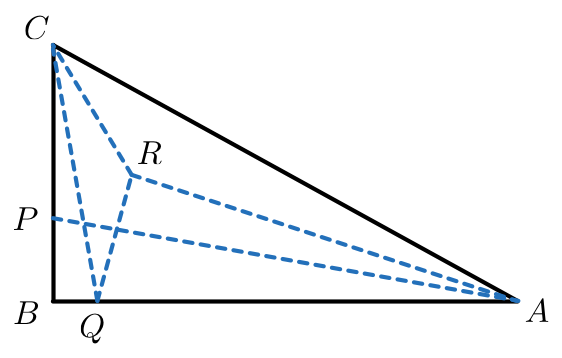# Angle Trisectors Of A Right Triangle

Geometry Level 5Triangle $ABC$ has angles $\angle A=3\alpha, \angle B=90^\circ$ and $\angle C=3\gamma$. Let $P$ be a point in segment $BC$ such that $\angle PAB=\alpha$, and $Q$ be a point in segment $BA$ such that $\angle QCB=\gamma$. Let $R$ be a point within the triangle such that $\angle RAC=\alpha$ and $\angle RCA=\gamma$. If $\angle QRC=142^\circ$, what is the measure (in degrees) of $\alpha$?

×